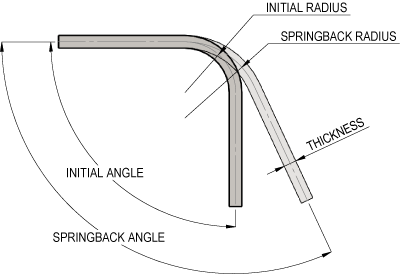## BENDING SPRINGBACK CALCULATOR

Springback occurs when a metal is bent and then tries to return to its original shape.

After a bending operation, residual stresses will cause the sheet metal to spring back slightly.

Due to this, it is necessary to over-bend the sheet an amount to acheive the desired bend radius and bend angle.

The final bend radius and bend angle can be approximated from the sheet thickness, K-factor, material yield strength and modulus of elasticity, and the initial bend angle and bend radius.

The final bend radius will be greater than initially formed and the final bend angle will be smaller.

The springback factor, commonly denoted by Ks, is the relation between the initial and final angles.

A springback factor of Ks = 1 means there is no springback, where a value of 0 means total springback.

We also have Air Bending Force, Bend Allowance, Deep Drawing Force and Wipe Bending Force Calculators.